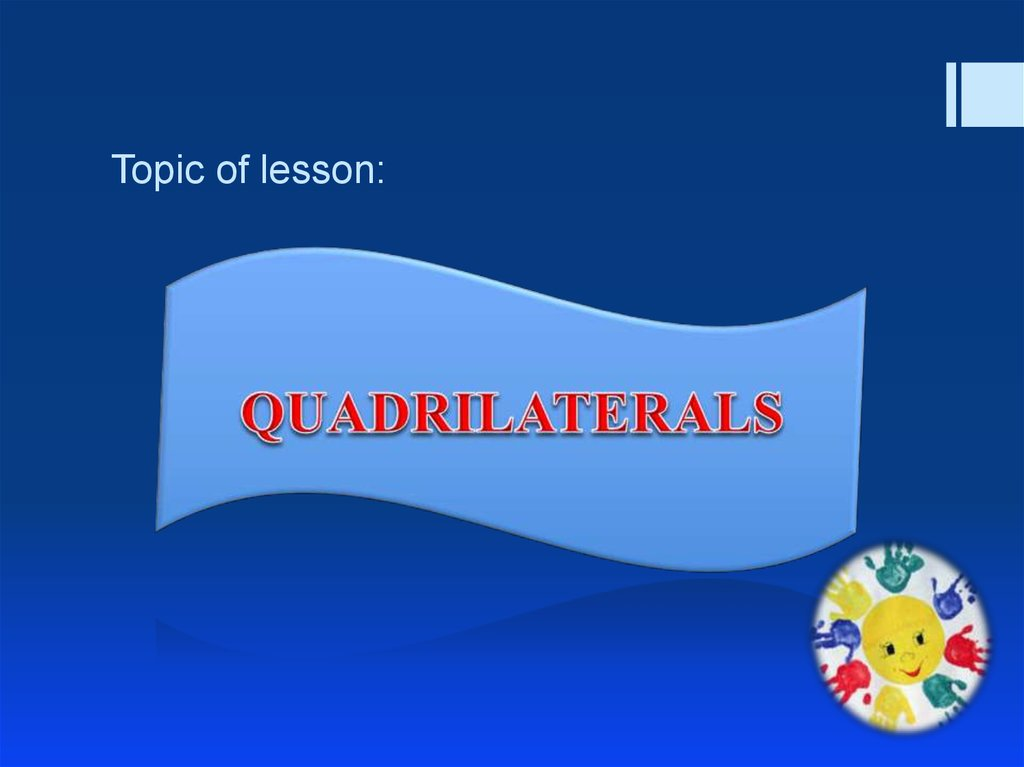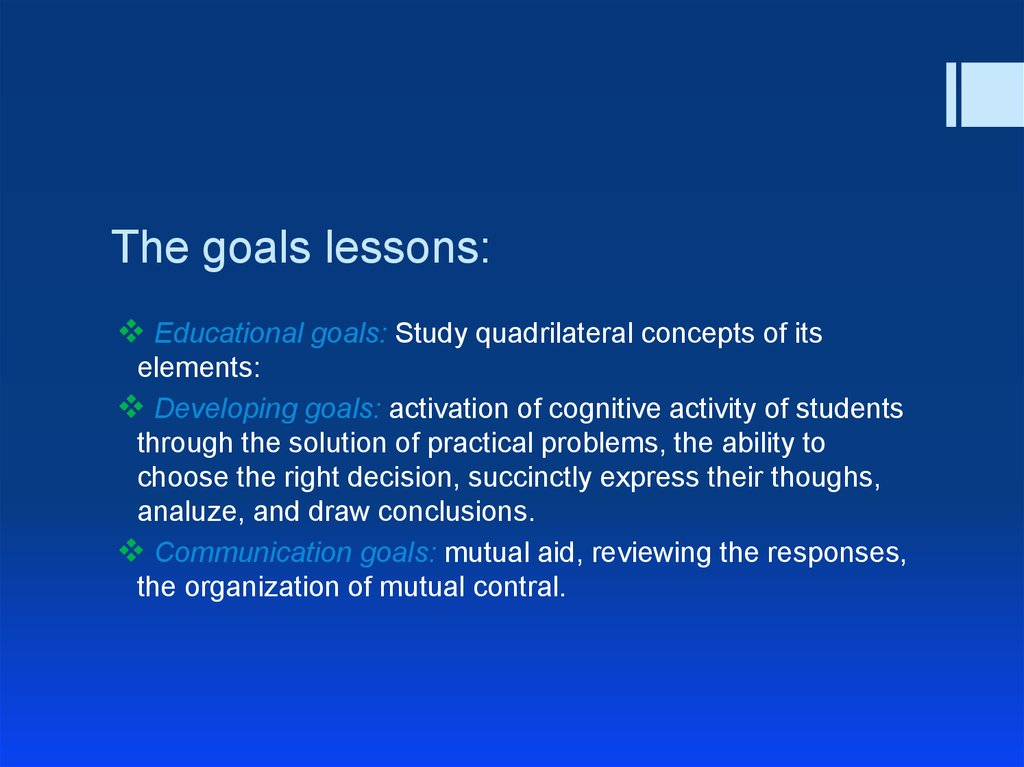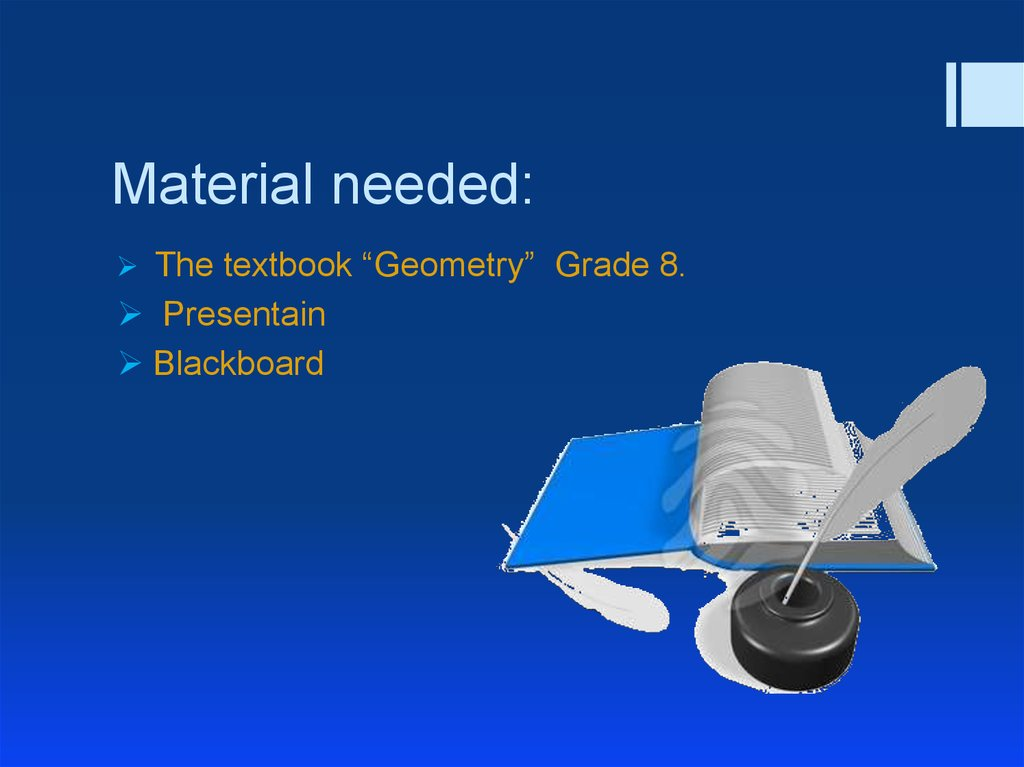## 2. The goals lessons:

Educational goals: Study quadrilateral concepts of its
elements:
Developing goals: activation of cognitive activity of students
through the solution of practical problems, the ability to
choose the right decision, succinctly express their thoughs,
analuze, and draw conclusions.
Communication goals: mutual aid, reviewing the responses,
the organization of mutual contral.

Presentain
Blackboard

## 4. Type of lesson:

Summarizing the lesson

## 5. Plan of lesson:

1. Topics include. (1-2min)
2. Сhecking homework. (4-5min)
3. Theoretical number material. (13-14min)
4. solving the problem. (20-23min)
5. HOMEWORK. (2-3min)

1
3
2
4
9
5
6
7
8
10
11
12
13
14

## 7. What is a tetragon (Quadrilateral)?

The Tetragon (Quadrilaterals) is a flat figure, which
consists their four lengths (segments),
consecutively connecting four points. No from
these point not to rest upon one direct, but
connecting their length (segments) are not crossed
(intersect).

## 8. That is a parallelogram?

The Parallelogram is a quadrilateral, the opposite sides being parallel two and
two (two by two parallel).
Name the characteristic of the sides and the angles of a parallelogram:
The opposite sides and angles of a parallelogram are equal.
Name the characteristic of the diagonals of a parallelogram.
The diagonals of a parallelogram are crossed (intersect) and divided fifty-fifty
cross point (into two equal parts by the point of their intersection).

## 9. types of parallelograms

The Rectangle - a parallelogram, beside which all corners
direct. Diagonals rectangle are.
The Rhombus - a parallelogram, beside which all sides are.
Diagonals of a rhombus are crossed under right angle and
are a bisections of its angles.
The Square - a rectangle, beside which all sides are.
Diagonals square are, are crossed under direct angle and
are a bisections of its angles.

В
А
А
В
О
О
Е
К
В
С
D
А
О
С
М

## 11. Solving problems.

- a parallelogram М + В = 144 о
Find the angles of the parallelogram.
1. Data: ABCМ
Solution. Since angle М and angle В opposite, that is 144 degrees
divided by 2 equals 72 degrees.
Similarly, angle A is equal to angle С.
But М + С = 180 о about angles С and D - two internal
unilateral corners, formed when crossing two parallel direct lines.
Then С = 180 о - М = 180 о – 72 о = 108 о.
The answer is: angle М is equal to angle В and makes 72 о, angle А
is equal to angle С and makes 108 o.

HOMEWORK
Page: 92-93
Exercise №5,7,14